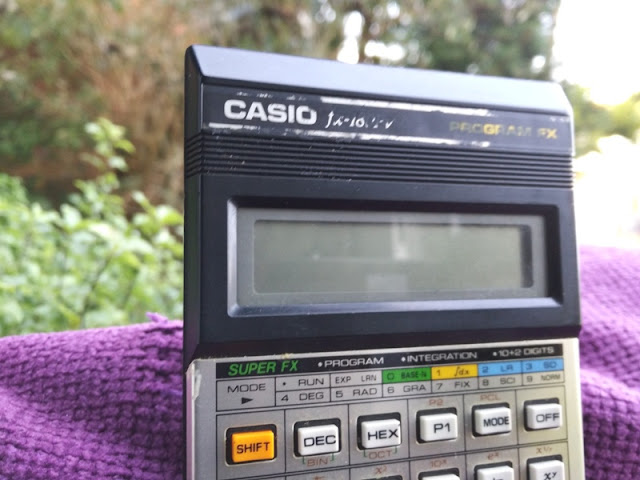The programmable calculator is another engineering wonder of the 1990's and the FX-180PV you see pictured is the one that saved me time and gave me piece of mind in my exams.This calculator was such a handy tool to have in class  and using them is not really cheating because you still have to know what you are doing to understand what the programmable calculator is telling you.The FX-180PV calculator is more than 20 years old, and I have just recently unearthed it from the same box of relics I found my original Game Boy handheld console.Programmable calculators were probably the closest thing you could get to a computer because like a computer, a programmable calculator can carry out in sequence user-given instructions.battery compartment for one single AA 1.5V battery
In this case, you can perform calculations in the same sequence as the written formula (true algebraic logic).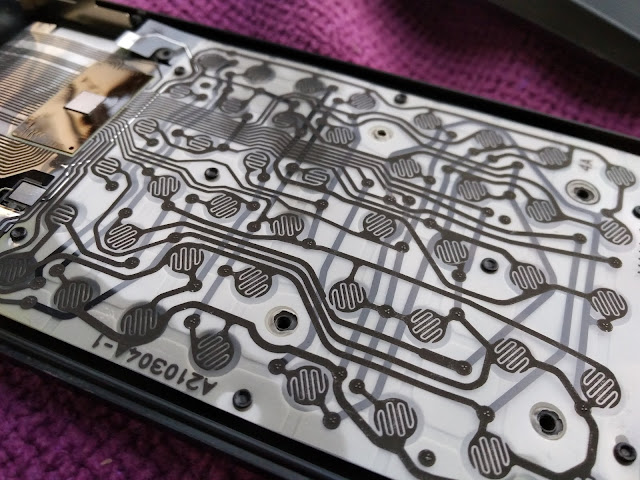Programmable calculators come pre-installed with a software  called Computer Algebra System (CAS) that allows you to carry out complicated mathematical calculations such as factor, expand, and simplify.With a programmable calculator such as the +CASIO FX-180PV, not only you can perform calculations like mathematicians and scientist do manually, but you can also store data in the symbolic form; hence why these types of calculators were referred to as "scientific calculators" since are used for solving calculations in the field of science, engineering and mathematics.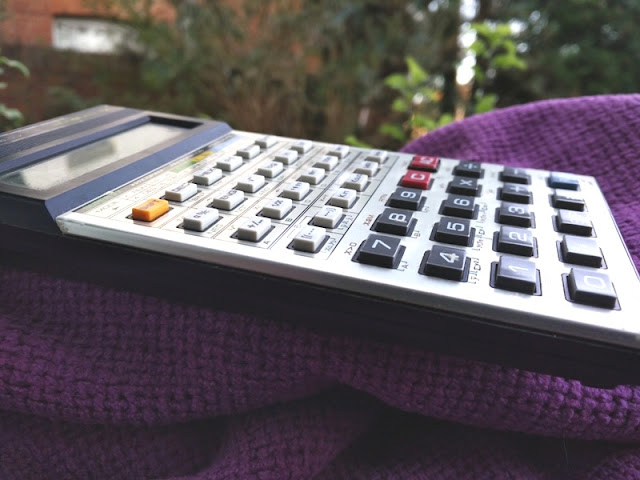As far as I know, when it comes to Casio calculators Casio programmable calculators feature the letter "P" in the model name; hence, the CASIO FX-180PV is a programmable calculator.
Casio's FX-180PV programmable scientific calculator has 6 constant memories and 1 independent memory, both of which are semi-non-volatile memory which means data stored doesn't get erased after turning off the calculator.two rubber feet on the bottom
With the FX-180PV, you can perform a total of 38 program steps and you can store up to two programmed procedures of calculations which is very convenient for repeating calculations with varying sets of data.The FX-180PV also had many built-in functions  including trigonometric/inverse trigonometric functions, common/natural logarithms, exponential functions, square roots, permutations, constant calculations, fraction calculations and change of base as well as statistical functions, integrals (Simpson's rule), fraction calculations and scientific functions.In total you get 12 modes of operation with the FX-180PV electronic calculator which are entered by pressing the "Mode" (yellow) button followed by a number from 0 to 9.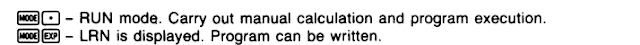The other two modes are RUN mode and LRN mode, which are entered by pressing Mode followed by "." and "exp" respectively. Run mode allows you to carry out manual calculation and program execution; while with LRN mode you can write a program.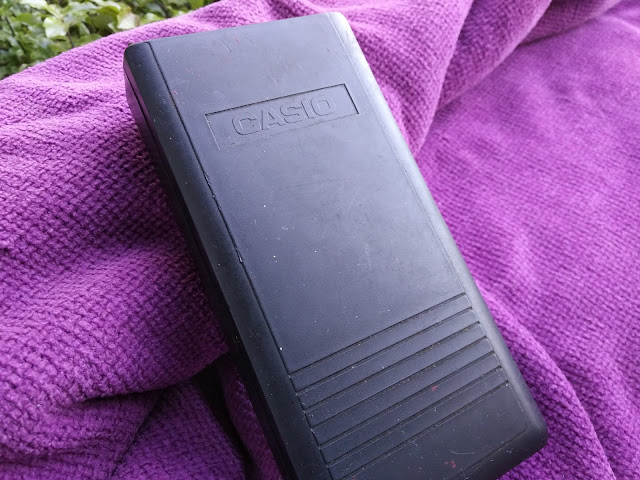protective case
As mentioned earlier, with the Casio's FX-180PV you can store calculations in the calculator's "independent memory" by pressing the Shift button and Min button. This function was great as it meant you could retrieve stored calculations even after turning off the calculator. As far as Binary/Octal/Decimal/Hexadecimal calculations and conversions, you can carry out these type of calculations in the BASE-N mode, which is entered by pressing Mode button and 0 button.

Let's see what else I can find in my treasure box of memories!